# Rolling In Still Water & Beam Swell

Q. What are the two main factors which govern still water rolling period?
Ans. T =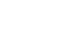T, the time period is independent of amplitude of roll for up to medium angles of roll. It mainly depends on Radius of gyration (and hence MI) & GMT.

1. If weights are placed away from axis of oscillation, the time period will increase.
2. T varies inversely as square root of initial metacentric height.

This relationship is similar to that provided by the formula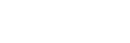which gives GMT varies inversely as square of true roll period.

Q. What will happen to rolling period if radius of gyration is increased two times and GM is increased four times?
Ans. The rolling period will not change. Since,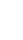is constant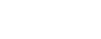new values are 2k & 4GM respectively.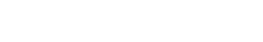Q. What is the rolling behavior in beam seas, when roll encounter period is smaller than the roll period of ship?
Ans.The influence of the wave system is represented by the expression:where T1 is the wave encounter period & T0 the ship’s own roll period. α is the maximum slope. The impact is negative when T1 < T0, because of the denominator becoming negative. The ship therefore, rolls in to the sea i.e. against the wave slope. In the figure below, θ is the angle viewed in inclinometer, whereas θ + φ is the roll angle (exaggerated impression) experienced on deck by a seaman.

Q. What is the rolling behavior in beam seas, when roll encounter period is bigger than the roll period of ship?
Ans. Referring to the same formula, the impact is positive when T1 > T0, because of the denominator becoming positive. The ship therefore, rolls away from the sea i.e. with the wave slope. In the figure below, θ is the angle viewed in inclinometer, whereas θ – φ is the roll angle (diminished impression) experienced on deck by a seaman.Q. How will you identify a synchronous rolling pattern?
Ans.Synchronous rolling will occur in beam seas, when the wave encounter period equals ship’s roll period. Thus, let us say, the ship is raised on crest and she rolls to starboard. The ship, when on trough will roll to port with increased amplitude. Similar, rolling pattern will continue with gradually increasing amplitude. Typical effect of the passage of each half wave length is to increase the forced amplitude of roll by a fixed amount depending on the maximum slope of wave. The roll amount increases in steps. Thus, if the step is of 6o, the roll angles will be 9, 15, 21, 27…. and so on. Theoretically, in the absence of resistance, the amplitude should build up until the ship capsizes. The increase in fixed steps, finally leading to a very large roll angle & then again starting from a very small angle is typical.Q. What avoiding action must be taken by the Captain?
Ans. Since, the synchronized rolling is caused due to the ship’s rolling getting synchronized with wave encounter period, the synchronism must be broken. This is done by either alteration of course or reduction of speed.

Q. If the wavelength of a wave is 122m, and the wave height is 4m, how will you find periodic time, maximum slope, velocity of the wave and also the wave encounter period?
Ans.Since, T2 = 2 π λ/g

If wavelength λ = 122m T1 = 8.829

Velocity of wave =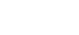=13.82m/sMaximum slope =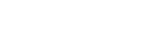= 0.102C = 5.8o

In a situation where the direction of wave propagation is 90o to the ship’s course, the time period of wave is wave encounter period. Thus, in this case it is 8.829sec.

Q. If above wave becomes synchronous, what will be the steps of increase in amplitude every half of the roll?
Ans. The size of step =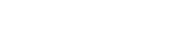.

Q. Now in above situation if own ship has natural rolling period 10sec and meets above wave system at 90o, what is the forced amplitude induced?
Ans. Amplitude of forced oscillation is:  =Q. What will be the wave encounter period if the course is altered by 50o?
Ans. If the course is altered by 50o, the angle between ship’s head and the direction of wave propagation (θ) will be 40O.

Hence,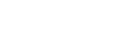All the speeds are in m/s. The + sign is for opposing directions of ship and wave.

Q. How does the forced amplitude change with the T1 – T0 ratio, in case of beam seas?
Ans. It can be very well explained with the help of a graph as shown below:

Thus, for an extremely small wave encounter period, the forced amplitude is very small too. The forced amplitude rises as the ratio approaches unity. For a very large value of the fraction T1 / T0, the forced amplitude becomes steady and is equal to slightly more than maximum slope. For T1 = T0, the forced amplitude tends to be very large (infinity).

(You may also visit my youtube videos @captsschaudhari.com)